# Estimate Orientation Through Inertial Sensor Fusion

This example shows how to use 6-axis and 9-axis fusion algorithms to compute orientation. There are several algorithms to compute orientation from inertial measurement units (IMUs) and magnetic-angular rate-gravity (MARG) units. This example covers the basics of orientation and how to use these algorithms.

### Orientation

An object's orientation describes its rotation relative to some coordinate system, sometimes called a parent coordinate system, in three dimensions.

For the following algorithms, the fixed, parent coordinate system used is North-East-Down (NED). NED is sometimes referred to as the global coordinate system or reference frame. In the NED reference frame, the X-axis points north, the Y-axis points east, and the Z-axis points downward. The X-Y plane of NED is considered to be the local tangent plane of the Earth. Depending on the algorithm, north may be either magnetic north or true north. The algorithms in this example use magnetic north.

If specified, the following algorithms can estimate orientation relative to East-North-Up (ENU) parent coordinate system instead of NED.

An object can be thought of as having its own coordinate system, often called the local or child coordinate system. This child coordinate system rotates with the object relative to the parent coordinate system. If there is no translation, the origins of both coordinate systems overlap.

The orientation quantity computed is a rotation that takes quantities from the parent reference frame to the child reference frame. The rotation is represented by a quaternion or rotation matrix.

### Types of Sensors

For orientation estimation, three types of sensors are commonly used: accelerometers, gyroscopes and magnetometers. Accelerometers measure proper acceleration. Gyroscopes measure angular velocity. Magnetometers measure the local magnetic field. Different algorithms are used to fuse different combinations of sensors to estimate orientation.

### Sensor Data

Through most of this example, the same set of sensor data is used. Accelerometer, gyroscope, and magnetometer sensor data was recorded while a device rotated around three different axes: first around its local Y-axis, then around its Z-axis, and finally around its X-axis. The device's X-axis was generally pointed southward for the duration of the experiment.

```ld = load('rpy_9axis.mat'); acc = ld.sensorData.Acceleration; gyro = ld.sensorData.AngularVelocity; mag = ld.sensorData.MagneticField; viewer = HelperOrientationViewer; ```

### Accelerometer-Magnetometer Fusion

The `ecompass` function fuses accelerometer and magnetometer data. This is a memoryless algorithm that requires no parameter tuning, but the algorithm is highly susceptible to sensor noise.

```qe = ecompass(acc, mag); for ii=1:size(acc,1) viewer(qe(ii)); pause(0.01); end ```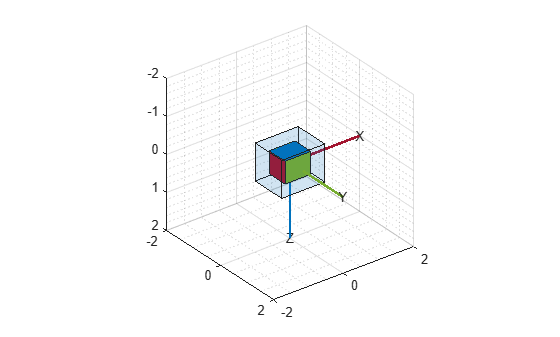Note that the `ecompass` algorithm correctly finds the location of north. However, because the function is memoryless, the estimated motion is not smooth. The algorithm could be used as an initialization step in an orientation filter or some of the techniques presented in the Lowpass Filter Orientation Using Quaternion SLERP could be used to smooth the motion.

### Accelerometer-Gyroscope Fusion

The following objects estimate orientation using either an error-state Kalman filter or a complementary filter. The error-state Kalman filter is the standard estimation filter and allows for many different aspects of the system to be tuned using the corresponding noise parameters. The complementary filter can be used as a substitute for systems with memory constraints, and has minimal tunable parameters, which allows for easier configuration at the cost of finer tuning.

The `imufilter` and `complementaryFilter` System objects™ fuse accelerometer and gyroscope data. The `imufilter` uses an internal error-state Kalman filter and the `complementaryFilter` uses a complementary filter. The filters are capable of removing the gyroscope's bias noise, which drifts over time.

```ifilt = imufilter('SampleRate', ld.Fs); for ii=1:size(acc,1) qimu = ifilt(acc(ii,:), gyro(ii,:)); viewer(qimu); pause(0.01); end ```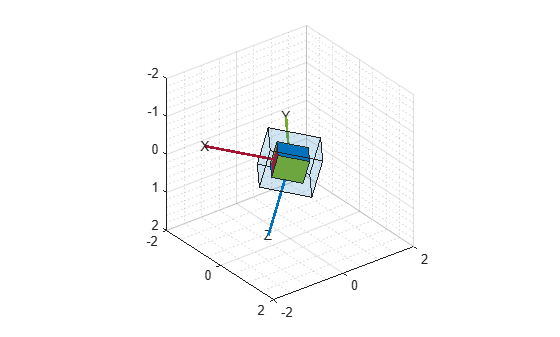```% Disable magnetometer input. cfilt = complementaryFilter('SampleRate', ld.Fs, 'HasMagnetometer', false); for ii=1:size(acc,1) qimu = cfilt(acc(ii,:), gyro(ii,:)); viewer(qimu); pause(0.01); end ```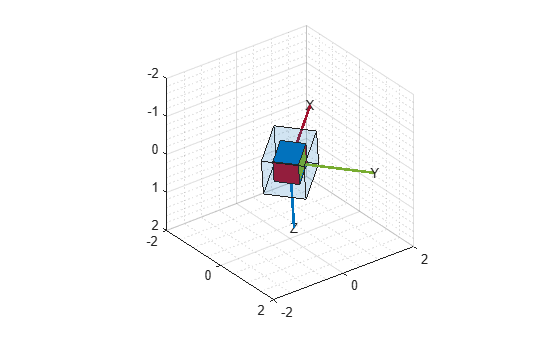Although the `imufilter` and `complementaryFilter` algorithms produce significantly smoother estimates of the motion, compared to the `ecompass`, they do not correctly estimate the direction of north. The `imufilter` does not process magnetometer data, so it simply assumes the device's X-axis is initially pointing northward. The motion estimate given by `imufilter` is relative to the initial estimated orientation. The `complementaryFilter` makes the same assumption when the `HasMagnetometer` property is set to `false`.

### Accelerometer-Gyroscope-Magnetometer Fusion

An attitude and heading reference system (AHRS) consists of a 9-axis system that uses an accelerometer, gyroscope, and magnetometer to compute orientation. The `ahrsfilter` and `complementaryFilter` System objects™ combine the best of the previous algorithms to produce a smoothly changing estimate of the device orientation, while correctly estimating the direction of north. The `complementaryFilter` uses the same complementary filter algorithm as before, with an extra step to include the magnetometer and improve the orientation estimate. Like `imufilter`, `ahrsfilter` algorithm also uses an error-state Kalman filter. In addition to gyroscope bias removal, the `ahrsfilter` has some ability to detect and reject mild magnetic jamming.

```ifilt = ahrsfilter('SampleRate', ld.Fs); for ii=1:size(acc,1) qahrs = ifilt(acc(ii,:), gyro(ii,:), mag(ii,:)); viewer(qahrs); pause(0.01); end ```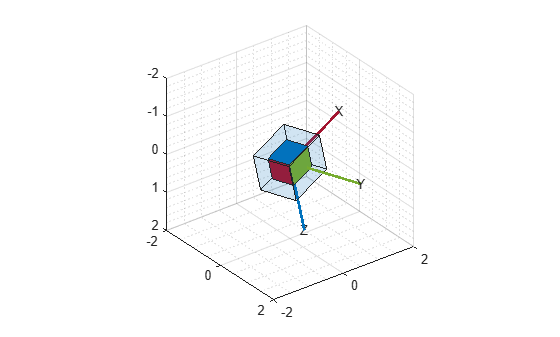```cfilt = complementaryFilter('SampleRate', ld.Fs); for ii=1:size(acc,1) qahrs = cfilt(acc(ii,:), gyro(ii,:), mag(ii,:)); viewer(qahrs); pause(0.01); end ```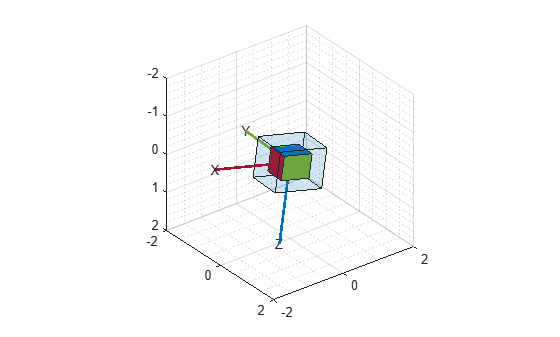### Tuning Filter Parameters

The `complementaryFilter`, `imufilter`, and `ahrsfilter` System objects™ all have tunable parameters. Tuning the parameters based on the specified sensors being used can improve performance.

The `complementaryFilter` parameters `AccelerometerGain` and `MagnetometerGain` can be tuned to change the amount each sensor's measurements impact the orientation estimate. When `AccelerometerGain` is set to `0`, only the gyroscope is used for the x- and y-axis orientation. When `AccelerometerGain` is set to `1`, only the accelerometer is used for the x- and y-axis orientation. When `MagnetometerGain` is set to `0`, only the gyroscope is used for the z-axis orientation. When `MagnetometerGain` is set to `1`, only the magnetometer is used for the z-axis orientation.

The `ahrsfilter` and `imufilter` System objects™ have more parameters that can allow the filters to more closely match specific hardware sensors. The environment of the sensor is also important to take into account. The `imufilter` parameters are a subset of the `ahrsfilter` parameters. The `AccelerometerNoise`, `GyroscopeNoise`, `MagnetometerNoise`, and `GyroscopeDriftNoise` are measurement noises. The sensors' datasheets help determine those values.

The `LinearAccelerationNoise` and `LinearAccelerationDecayFactor` govern the filter's response to linear (translational) acceleration. Shaking a device is a simple example of adding linear acceleration.

Consider how an `imufilter` with a `LinearAccelerationNoise` of 9e-3responds to a shaking trajectory, compared to one with a `LinearAccelerationNoise` of 9e-4.

```ld = load('shakingDevice.mat'); accel = ld.sensorData.Acceleration; gyro = ld.sensorData.AngularVelocity; viewer = HelperOrientationViewer; highVarFilt = imufilter('SampleRate', ld.Fs, ... 'LinearAccelerationNoise', 0.009); qHighLANoise = highVarFilt(accel, gyro); lowVarFilt = imufilter('SampleRate', ld.Fs, ... 'LinearAccelerationNoise', 0.0009); qLowLANoise = lowVarFilt(accel, gyro); ```

One way to see the effect of the `LinearAccelerationNoise` is to look at the output gravity vector. The gravity vector is simply the third column of the orientation rotation matrix.

```rmatHigh = rotmat(qHighLANoise, 'frame'); rmatLow = rotmat(qLowLANoise, 'frame'); gravDistHigh = sqrt(sum( (rmatHigh(:,3,:) - [0;0;1]).^2, 1)); gravDistLow = sqrt(sum( (rmatLow(:,3,:) - [0;0;1]).^2, 1)); figure; plot([squeeze(gravDistHigh), squeeze(gravDistLow)]); title('Euclidean Distance to Gravity'); legend('LinearAccelerationNoise = 0.009', ... 'LinearAccelerationNoise = 0.0009'); ```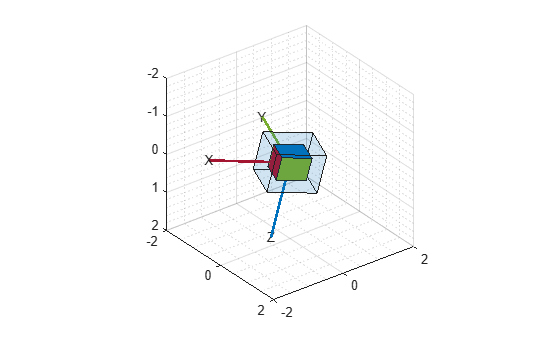The `lowVarFilt` has a low `LinearAccelerationNoise`, so it expects to be in an environment with low linear acceleration. Therefore, it is more susceptible to linear acceleration, as illustrated by the large variations earlier in the plot. However, because it expects to be in an environment with a low linear acceleration, higher trust is placed in the accelerometer signal. As such, the orientation estimate converges quickly back to vertical once the shaking has ended. The converse is true for `highVarFilt`. The filter is less affected by shaking, but the orientation estimate takes longer to converge to vertical when the shaking has stopped.

The `MagneticDisturbanceNoise` property enables modeling magnetic disturbances (non-geomagnetic noise sources) in much the same way `LinearAccelerationNoise` models linear acceleration.

The two decay factor properties (`MagneticDisturbanceDecayFactor` and `LinearAccelerationDecayFactor`) model the rate of variation of the noises. For slowly varying noise sources, set these parameters to a value closer to 1. For quickly varying, uncorrelated noises, set these parameters closer to 0. A lower `LinearAccelerationDecayFactor` enables the orientation estimate to find "down" more quickly. A lower `MagneticDisturbanceDecayFactor` enables the orientation estimate to find north more quickly.

Very large, short magnetic disturbances are rejected almost entirely by the `ahrsfilter`. Consider a pulse of [0 250 0] uT applied while recording from a stationary sensor. Ideally, there should be no change in orientation estimate.

```ld = load('magJamming.mat'); hpulse = ahrsfilter('SampleRate', ld.Fs); len = 1:10000; qpulse = hpulse(ld.sensorData.Acceleration(len,:), ... ld.sensorData.AngularVelocity(len,:), ... ld.sensorData.MagneticField(len,:)); figure; timevec = 0:ld.Fs:(ld.Fs*numel(qpulse) - 1); plot( timevec, eulerd(qpulse, 'ZYX', 'frame') ); title(['Stationary Trajectory Orientation Euler Angles' newline ... 'Magnetic Jamming Response']); legend('Z-rotation', 'Y-rotation', 'X-rotation'); ylabel('Degrees'); xlabel('Seconds'); ```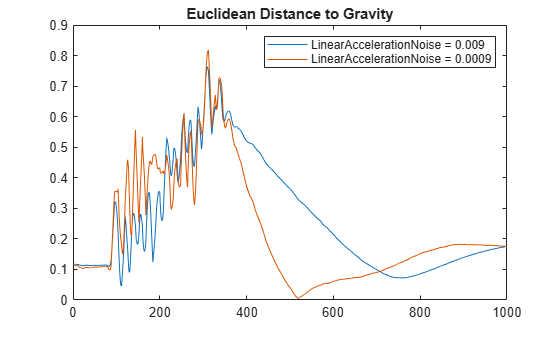Note that the filter almost totally rejects this magnetic pulse as interference. Any magnetic field strength greater than four times the `ExpectedMagneticFieldStrength` is considered a jamming source and the magnetometer signal is ignored for those samples.

### Conclusion

The algorithms presented here, when properly tuned, enable estimation of orientation and are robust against environmental noise sources. It is important to consider the situations in which the sensors are used and tune the filters accordingly.

##### SupportGet trial now